# 楚雄市建成区道路两侧垃圾桶分布及优化研究Research on Distribution and Optimization of Garbage Bins on Both Sides of Road in Built Area of Chuxiong City

DOI: 10.12677/GSER.2020.93019, PDF, HTML, XML, 下载: 157  浏览: 1,148  科研立项经费支持

Abstract: The number and distribution of road garbage bins have an important relationship with the urban appearance construction, and also have an important significance for improving the utilization rate of resources. This paper investigates the types and distribution of trash bins on both sides of the road in the built-up area of Chuxiong City, and uses the average center, median center, center elements, and direction distribution methods to evaluate the distribution of trash bins. The results showed that the sanitation trash bins on both sides of the main road are less distributed, there are not enough garbage bins on both sides of the eastern fringe road, the overall number and direction of trash bins are unevenly distributed, the number of garbage transfer boxes and sanitation garbage bins is small and they are unevenly distributed. According to the existing problems, this paper made the following suggestions: three types of trash bins should be set in areas where residents live, the number of garbage bins should refer to the density of residents, garbage transfer box and sanitation garbage box should be placed according to the standard, the trash bins should be set in a prominent position.

1. 引言

2. 研究区概况

3. 研究方法

$\stackrel{¯}{X}\text{=}\frac{\underset{i=1}{\overset{n}{\sum }}Xi}{n},\text{\hspace{0.17em}}\text{\hspace{0.17em}}\stackrel{¯}{Y}=\frac{\underset{i=1}{\overset{n}{\sum }}Yi}{n}$

$\text{\hspace{0.17em}}{d}_{i}^{t}=\sqrt{{\left({X}_{i}-{X}^{t}\right)}^{2}+{\left({Y}_{i}-{Y}^{t}\right)}^{2}}$

$SD{E}_{x}=\sqrt{\frac{\underset{i=1}{\overset{n}{\sum }}{\left({x}_{i}-\stackrel{¯}{X}\right)}^{2}}{n}}$

$SD{E}_{y}=\sqrt{\frac{\underset{i=1}{\overset{n}{\sum }}{\left({y}_{i}-\stackrel{¯}{Y}\right)}^{2}}{n}}$

$\mathrm{tan}\theta =\frac{A+B}{C}$

$A=\left(\underset{i=1}{\overset{n}{\sum }}{\stackrel{˜}{x}}_{i}^{2}-\underset{i=1}{\overset{n}{\sum }}{\stackrel{˜}{y}}_{i}^{2}\right)$

${\sigma }_{x}=\sqrt{2}\sqrt{\frac{\underset{i=1}{\overset{n}{\sum }}{\left({\stackrel{˜}{x}}_{i}\mathrm{cos}\theta -\stackrel{˜}{y}\mathrm{sin}\theta \right)}^{2}}{n}}$

${\sigma }_{y}=\sqrt{2}\sqrt{\frac{\underset{i=1}{\overset{n}{\sum }}{\left({\stackrel{˜}{x}}_{i}\mathrm{sin}\theta +{\stackrel{˜}{y}}_{i}\mathrm{cos}\theta \right)}^{2}}{n}}$

4. 研究结果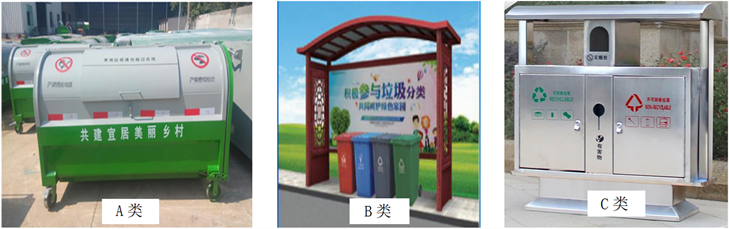Figure 1. Three kinds of garbage can

1) A类垃圾桶分布情况

A类垃圾桶总体数量较少，空间分布稀疏，多是沿街分布，楚雄市建成区西南和西北方向因商铺小区分布较为密集，A类垃圾桶错落分布在其中间，但楚雄市建成区中部区域分布数量较少且分布密度低(图2)。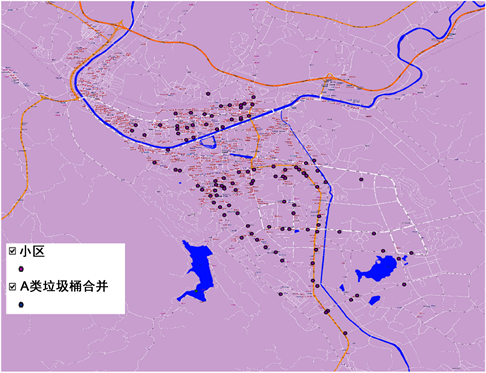Figure 2. Distribution of class A garbage cans

2) B类垃圾桶分布情况

B类垃圾桶总体数量不多，多数是以一定间隔沿道路分布在两侧，小区及商业街内部分布较少，但总体位置分布较全面，但数量不足(图3)。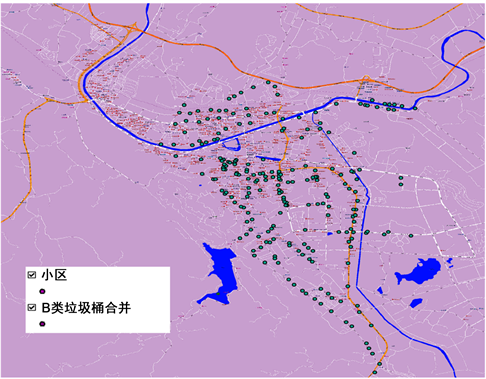Figure 3. Distribution of type B garbage cans

3) C类垃圾桶分布情况

C类垃圾桶沿道路两侧分布，数量众多，空间分布密度大，多数C类垃圾桶分布在道路两侧，小区内部数量较少(图4)。Figure 4. Distribution of C type garbage cans

4) ABC三类垃圾桶分布汇总情况(图5)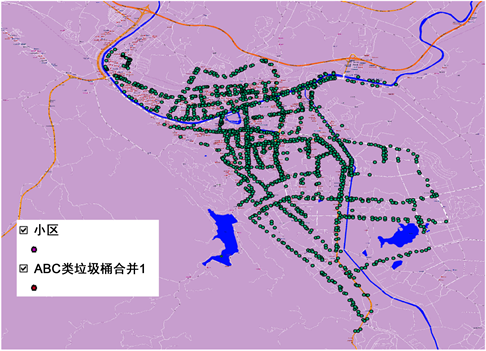Figure 5. Classification of three types of garbage cans

5) 平均中心分布情况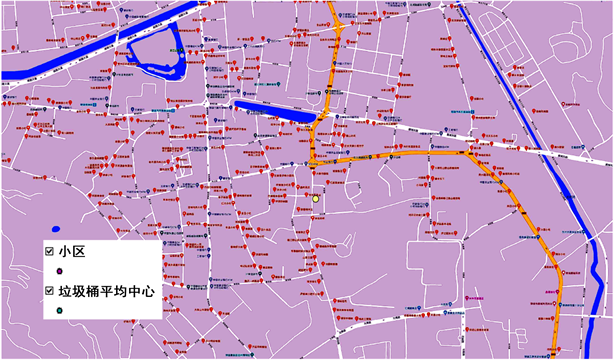Figure 6. Average center distribution of garbage cans

6) 中位数中心分布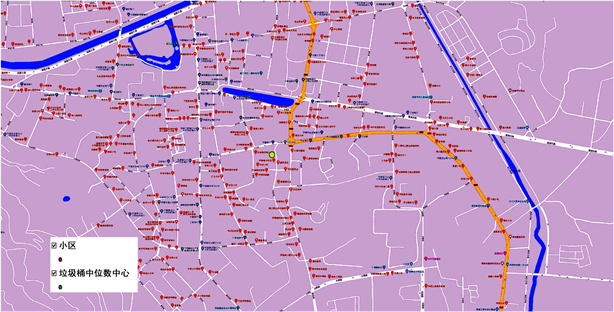Figure 7. Distribution of median center of garbage cans

7) 中心要素分布情况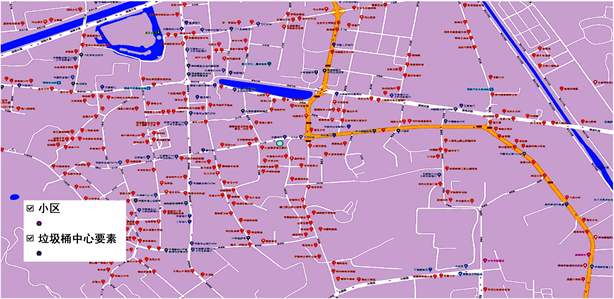Figure 8. Distribution of garbage bin center elements

8) 方向分布情况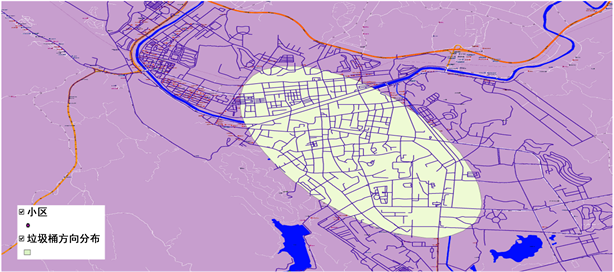Figure 9. Distribution of garbage cans

5. 结论与建议

5.1. 楚雄市建成区道路两侧垃圾桶分布现状合理性分析

5.2. 楚雄市建成区道路两侧垃圾桶分布现状不足之处

5.3. 对楚雄市建成区道路两侧垃圾桶分布的改进建议

NOTES

*通讯作者。

  中华人民共和国建设部. GB50337-2003城市环境卫生设施规划规范[S]. 北京: 中国建筑工业出版社, 2003.  李建辉, 陈慧. 基于GIS的黄河水院校园垃圾桶优化配置研究[J]. 黄河水利职业技术学院学报, 2014, 26(1): 41-43.  黄周满, 尹春燕, 李明. 校园垃圾桶的分布调查与分析——以武昌理工学院为例[J]. 现代交际, 2014(4): 56, 57.  卢绪凯. 垃圾桶分布的调查分析及其对垃圾回收的影响[J]. 资源节约与环保, 2019(6): 124.  徐玉莹, 秦宇幸, 赵安琪, 高翔. 中国海洋大学校园垃圾桶分布的数学模型研究[J]. 运筹与模糊学, 2017, 7(1): 10-21.  宗会明, 杨夏夏. 基于城市功能区的道路垃圾桶布局研究——以重庆市北碚城区为例[J]. 西南大学学报(自然科学版), 2014(10): 124-129.  陈姗姗. 基于政府监管视角的垃圾回收现状与对策研究[J]. 再生资源与循环经济, 2016, 9(12): 20-23.